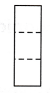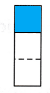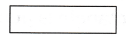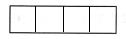# McGraw Hill Math Grade 2 Chapter 7 Lesson 5 Answer Key Dividing Rectangles into Equal Parts

Practice questions available in McGraw Hill Math Grade 2 Answer Key PDF Chapter 7 Lesson 5 Dividing Rectangles into Equal Parts will engage students and is a great way of informal assessment.

## McGraw-Hill Math Grade 2 Answer Key Chapter 7 Lesson 5 Dividing Rectangles into Equal Parts

Make Equal Parts

Question 1.
Color half of the rectangle.Explanation:
I colored the half of the rectangle.

Question 2.
Color a third of the rectangle.Explanation:
I colored a third that is 1 part of 3 parts of the rectangle.

Question 3.
Draw lines to divide the rectangle into fourths.Explanation:
I drew lines to divide the rectangle into fourths that is 4 parts.

Question 4.
Circle the rectangle that shows thirds.